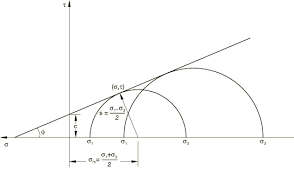# How to Calculate and Solve for Mohr – Coulomb Criterion | Rock MechanicsThe image above represents mohr – coulomb criterion.

To compute for mohr – coulomb criterion, three essential parameters are needed and these parameters are Cohesion (|τo), Co-efficient of Friction (μ) and Normal Stress (σn).

The formula for calculating the mohr – coulomb criterion:

|τ| = τo + μσn

Where;

|τ = Mohr – Coulomb Criterion
o = Cohesion
μ = Co-efficient of Friction
σn = Normal Stress

Let’s solve an example;
Find the mohr – coulomb criterion when the cohesion is 7, the co-efficient of friction is 30 and the normal stress is 28.

This implies that;

o = Cohesion = 7
μ = Co-efficient of Friction = 30
σn = Normal Stress = 28

|τ| = τo + μσn
|τ| = 7 + 30(28)
|τ| = 7 + 840
|τ| = 847

Therefore, the mohr – coulomb criterion is 847.

Calculating the Cohesion when the Mohr – Coulomb Criterion, the Co-efficient of Friction and the Normal Stress is Given.

o = |τ|  – μσn

Where;

o = Cohesion
|τ = Mohr – Coulomb Criterion
μ = Co-efficient of Friction
σn = Normal Stress

Let’s solve an example;
Find the cohesion when the mohr – coulomb criterion is 44, the co-efficient of friction is 10 and the normal stress is 4.

This implies that;

|τ = Mohr – Coulomb Criterion = 44
μ = Co-efficient of Friction = 10
σn = Normal Stress = 4

o = |τ|  – μσn
o = 44  – 10(4)
o = 44  – 40
o = 4

Therefore, the cohesion is 4.

Calculating the Co-efficient of Friction when the Mohr – Coulomb Criterion, the Cohesion and the Normal Stress is Given.

μ = |τ| – |τo / σn

Where;

μ = Co-efficient of Friction
|τ = Mohr – Coulomb Criterion
o = Cohesion
σn = Normal Stress

Let’s solve an example;
Find the co-efficient of friction when the cohesion is 10, the mohr – coulomb criterion is 24 and the normal stress is 12.

This implies that;

|τ = Mohr – Coulomb Criterion = 24
o = Cohesion = 10
σn = Normal Stress = 12

μ = |τ| – |τo / σn
μ = 24 – 10 / 12
μ = 14 / 12
μ = 1.667

Therefore, the co-efficient of friction is 1.667.

Calculating the Normal Stress when the Mohr – Coulomb Criterion, the Co-efficient of Friction and the Cohesion is Given.

σn = |τ| – |τo / μ

Where;

σn = Normal Stress
|τ = Mohr – Coulomb Criterion
o = Cohesion
μ = Co-efficient of Friction

Let’s solve an example;
Find the normal stress when the mohr – coulomb criterion is 30, the cohesion is 14 and the co-efficient of friction is 5.

This implies that;

|τ = Mohr – Coulomb Criterion = 30
o = Cohesion = 14
μ = Co-efficient of Friction = 5

σn = |τ| – |τo / μ
σn = 30 – 14 / 5
σn = 16 / 5
σn = 3.2

Therefore, the normal stress is 3.2.

Nickzom Calculator – The Calculator Encyclopedia is capable of calculating the mohr – coulomb criterion.

To get the answer and workings of the mohr – coulomb criterion using the Nickzom Calculator – The Calculator Encyclopedia. First, you need to obtain the app.

You can get this app via any of these means:

To get access to the professional version via web, you need to register and subscribe for NGN 1,500 per annum to have utter access to all functionalities.
You can also try the demo version via https://www.nickzom.org/calculator

Apple (Paid) – https://itunes.apple.com/us/app/nickzom-calculator/id1331162702?mt=8
Once, you have obtained the calculator encyclopedia app, proceed to the Calculator Map, then click on Geology under Add-on.Now, Click on Rock Mechanics under GeologyNow, Click on Mohr – Coulomb Criterion under Rock MechanicsThe screenshot below displays the page or activity to enter your values, to get the answer for the mohr – coulomb criterion according to the respective parameters which are the Cohesion (|τo), Co-efficient of Friction (μ) and Normal Stress (σn).Now, enter the values appropriately and accordingly for the parameters as required by the Cohesion (|τo) is 7, Co-efficient of Friction (μ) is 30 and Normal Stress (σn) is 28.Finally, Click on CalculateAs you can see from the screenshot above, Nickzom Calculator– The Calculator Encyclopedia solves for the mohr – coulomb criterion and presents the formula, workings and steps too.